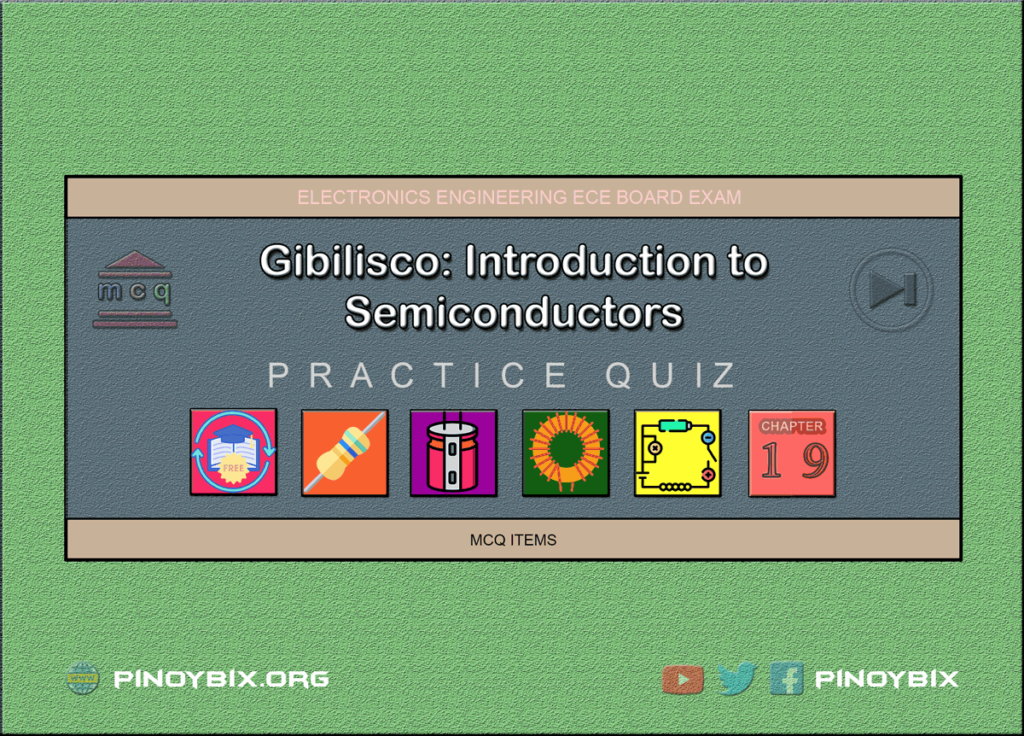# Gibilisco: MCQ in Introduction to Semiconductors | ECE Board Exam

(Last Updated On: April 29, 2022)This is the Multiple Choice Questions (MCQs) in Chapter 19: Introduction to Semiconductors from the book Teach Yourself Electricity and Electronics, 5th edition by Stan Gibilisco. If you are looking for a reviewer in Electronics Engineering this will definitely help you before taking the Board Exam.

Start Practice Exam Test Questions

Choose the letter of the best answer in each questions.

1. The term “semiconductor” arises from:

A. Resistor-like properties of metal oxides.

B. Variable conductive properties of some materials.

C. The fact that there’s nothing better to call silicon.

D. Insulating properties of silicon and GaAs.

Solution:

2. Which of the following is not an advantage of semiconductor devices over vacuum tubes?

A. Smaller size.

B. Lower working voltage.

C. Lighter weight.

D. Ability to withstand high voltages.

Solution:

3. The most common semiconductor among the following substances is:

A. Germanium.

B. Galena.

C. Silicon.

D. Copper.

Solution:

4. GaAs is a(n):

A. Compound.

B. Element.

C. Conductor.

D. Gas.

Solution:

5. A disadvantage of gallium-arsenide devices is that:

A. The charge carriers move fast.

B. The material does not react to ionizing radiation.

C. It is expensive to produce.

D. It must be used at high frequencies.

Solution:

6. Selenium works especially well in:

A. Photocells.

B. High-frequency detectors.

D. Voltage regulators.

Solution:

7. Of the following, which material allows the lowest forward voltage drop in a diode?

A. Selenium.

B. Silicon.

C. Copper.

D. Germanium.

Solution:

8. A CMOS integrated circuit:

A. Can only work at low frequencies.

B. Is susceptible to damage by static.

C. Requires considerable power to function.

D. Needs very high voltage.

Solution:

9. The purpose of doping is to:

A. Make the charge carriers move faster.

B. Cause holes to flow.

C. Give a semiconductor material certain properties.

D. Protect devices from damage in case of transients.

Solution:

10. A semiconductor material is made into N type by:

C. Injecting electrons.

D. Taking electrons away.

Solution:

11. Which of the following does not result from adding an acceptor impurity?

A. The material becomes P type.

B. Current flows mainly in the form of holes.

C. Most of the carriers have positive electric charge.

D. The substance has an electron surplus.

Solution:

12. In a P-type material, electrons are:

A. Majority carriers.

B. Minority carriers.

C. Positively charged.

D. Entirely absent.

Solution:

13. Holes flow from:

A. Minus to plus.

B. Plus to minus.

C. P-type to N-type material.

D. N-type to P-type material.

Solution:

14. When a P-N junction does not conduct, it is:

A. Reverse biased.

B. Forward biased.

C. Biased past the breaker voltage.

D. In a state of avalanche effect.

Solution:

15. Holes flow the opposite way from electrons because:

A. Charge carriers flow continuously.

B. Charge carriers are passed from atom to atom.

C. They have the same polarity.

D. No! Holes flow in the same direction as electrons.

Solution:

16. If an electron has a charge of -1 unit, a hole has:

A. A charge of -1 unit.

B. No charge.

C. A charge of +1 unit.

D. A charge that depends on the semiconductor type.

Solution:

17. When a P-N junction is reverse-biased, the capacitance depends on all of the following except:

A. The frequency.

B. The width of the depletion region.

C. The cross-sectional area of the junction.

D. The type of semiconductor material.

Solution:

18. If the reverse bias exceeds the avalanche voltage in a P-N junction:

A. The junction will be destroyed.

B. The junction will insulate; no current will flow.

C. The junction will conduct current.

D. The capacitance will become extremely high.

Solution:

19. Avalanche voltage is routinely exceeded when a P-N junction acts as:

A. Current rectifier.

B. Variable resistor.

C. Variable capacitor.

D. Voltage regulator.

Solution:

20. An unimportant factor concerning the frequency at which a P-N junction will work effectively is:

A. The type of semiconductor material.

B. The cross-sectional area of the junction.

C. The reverse current.

D. The capacitance with reverse bias.

Solution:

### Topics Included in the Test from Chapter 19 to Chapter 30

Part 3: Basic Electronics
Chapter 24: MCQ in Amplifiers
Chapter 25: MCQ in Oscillators

### Complete List of Multiple Choice Questions from this Book

1.Priel June
2.Priel June
PinoyBIX Engineering. © 2014-2021 All Rights Reserved | How to Donate? |